# Definition | 3D Geometry Concepts | Cube

This is a collection of definitions related to 3D figures and their properties. This includes definitions and examples of 2D nets of 3D figures, as well as 2D cross-sections of different 3D figures.

### To see the complete collection of definitions on this topic, click on this link.

A cube is an example of a Platonic Solid. Below we also include information about Platonic solids and 2D nets of these 3D figures. To get a better understanding of these 3D figures, study these basic forms.

Learn about the Platonic Solids by watching this video. The Platonic Solids include the cube, the tetrahedron, the octahedron, the icosahedron, and the dodecahedron. Each figure is analyzed with a two-dimensional net and then the three-dimensional figure. (Note: The video transcript is also included.)

## 3D Geometry: The Platonic Solids

Learn about the basics of 3D Geometry by learning about the Platonic Solids.Media4Math.com has thousands of digital resources for math.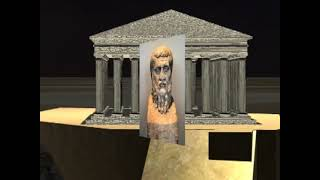The video was uploaded on 9/13/2022.

You can view the video here.

The video lasts for 3 minutes and 3 seconds.

Video Transcript

IN ANCIENT GREECE THE PHILOSOPHER PLATO DESCRIBED A SET OF THREE DIMENSIONAL SHAPES THAT HAVE SINCE COME TO BEAR HIS NAME: THE PLATONIC SOLIDS.

LET'S START WITH THEIR TWO DIMENSIONAL COUNTERPARTS AND BUILD THEIR THREE DIMENSIONAL VERSION.

LOOK AT THIS EQUILATERAL TRIANGLE. IT IS A REGULAR POLYGON WHERE ALL SIDES AND ANGLE MEASURES ARE CONGRUENT. THIS TWO DIMENSIONAL NET WHEN FOLDED THIS WAY BECOMES THE THREE DIMENSIONAL SOLID CALLED A TETRAHEDRON, WHICH IS ONE OF THE PLATONIC SOLIDS. BECAUSE OF THE UNDERLYING REGULAR POLYGON, THE TETRAHEDRON HAS CONGRUENT EDGES, VERTICES AND ANGLES.

NOW LOOK AT THIS SQUARE, WHICH IS A REGULAR QUADRILATERAL. WE USE THAT SQUARE TO CONSTRUCT A TWO DIMENSIONAL NET. WE FOLD THIS NET TO CONSTRUCT A CUBE, WHICH IS ANOTHER PLATONIC SOLID ALSO REFERRED TO AS A HEXAHEDRON. THE "HEX" IN HEXAHEDRON REFERS TO SIX, WHICH IS THE NUMBER OF SIDES IN A CUBE. LIKE THE TETRAHEDRON THE EDGES, VERTICES AND ANGLES OF THE CUBE ARE CONGRUENT TO EACH OTHER.

THE OTHER PLATONIC SOLIDS INCLUDE THE EIGHT-FACED OCTAHEDRON...

THE TWELVE-FACED DODECAHEDRON...

AND THE TWENTY-FACED ICOSAHEDRON.

WITH ALL THESE FIGURES, THE UNDERLYING REGULAR POLYGON SHAPE ENSURES CONGRUENT EDGES, ANGLES AND VERTICES.

BEYOND THE PLATONIC SOLIDS ARE MANY DIFFERENT THREE DIMENSIONAL SHAPES. IN THIS PROGRAM YOU WILL EXPLORE THE PROPERTIES OF THREE DIMENSIONAL FIGURES.

UNDERSTANDING THE PROPERTIES OF THESE FIGURES HELPS US UNDERSTAND CERTAIN NATURAL AND MAN-MADE STRUCTURES THAT SHARE THESE PROPERTIES.

IN PARTICULAR, THIS PROGRAM WILL COVER THE FOLLOWING KEY CONCEPTS:

## Properties of 3D Figures

Now that you have seen the video about the Platonic Solids, let's investigate three-dimensional figures in more detail. These figures have certain properties that can be analyzed and categorized.

This is a cube. It is a Platonic Solid, also known as a hexahedron.The surface of the cube is made up of six faces. Each of the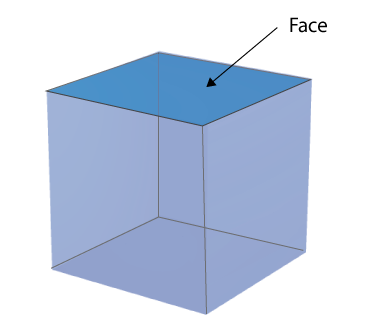Where the faces intersect is known as an edge. A cube has twelve edges. Do you see the twelve edges of this cube?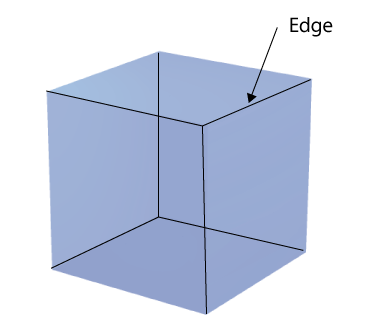The corners of a three-dimensional figure are known as vertices. A single corner is called a vertex. A cube has eight vertices. Do you see the eight vertices of this cube?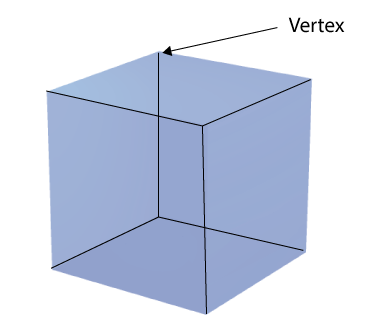Different three-dimensional figures have different numbers of faces, edges, and vertices. Here is a square pyramid. Do you see the square base of this pyramid? Do you see the four triangular faces?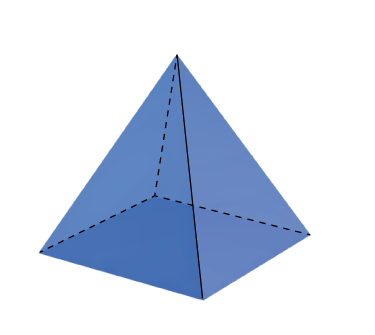The square pyramid has 4 faces, 8 edges, and 5 vertices.

Here is an octahedron, which is another of the Platonic solids. The octahedron is like two square pyramids attached at the base.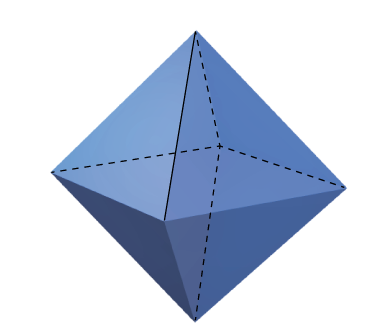A square pyramid has 8 faces, 12 edges, and 6 vertices.

This table summarizes these properties for a number of different three-dimensional shapes. This table identifies the three-dimensional figure and tracks the total number of faces, edges, and vertices.

 Figure Name Faces Edges Vertices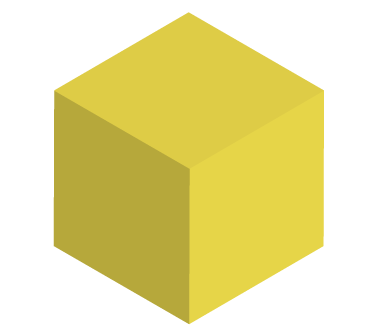Cube 6 12 8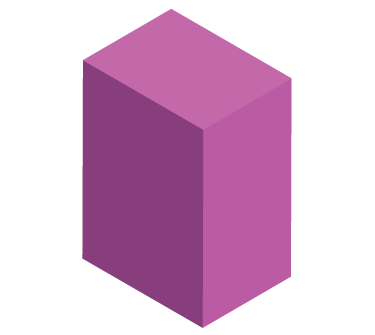Rectangular Prism 6 12 8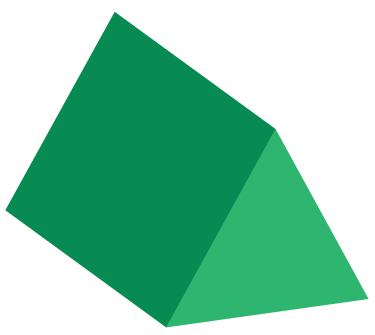Triangular Prism 5 9 6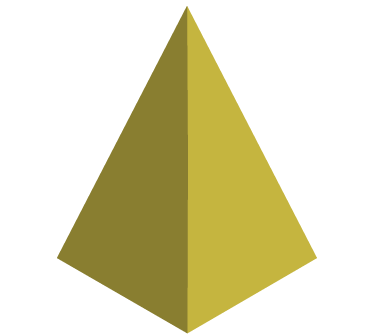Square Pyramid 5 8 5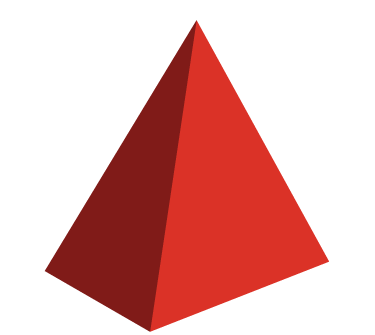Triangular Pyramid 4 6 4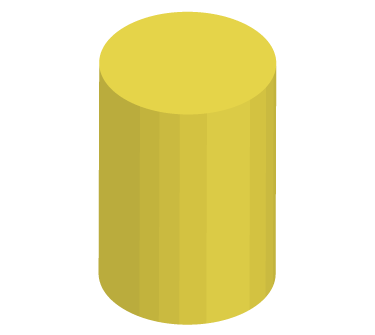Cylinder 2, plus 1 curved surface 2 0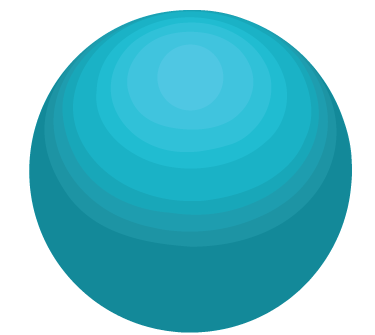Sphere 1 curved surface 0 0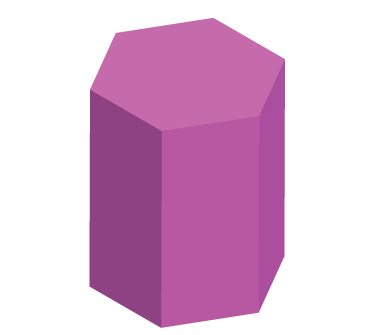Hexagonal Prism 8 18 12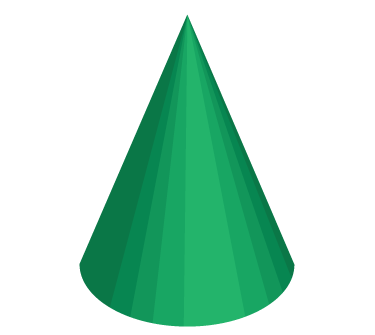Cone 1, plus 1 curved surface 1 1

## Using Nets to Understand 3D Figures

### What Is a Net?

Now that you have seen these different three-dimensional figures, did you notice many of them had two-dimensional faces? For example, a cube has eight square faces, while a cylinder has two circular sides.

### Many three-dimensional figures consist of two-dimensional part. In fact, with many 3D figures, we can break them down into 2D components. This can be done by using a net.

A net is a two-dimensional version of a three-dimensional figure. A printed net can be used to build the 3D model. Here are some examples of nets.

This is the net for a cube. Since each face of the cube is a square, the net for a cube consists of a series of squares that fold into a cube.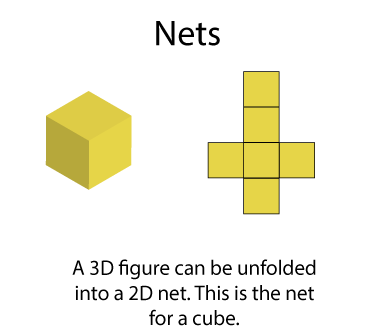This is the net for a triangular prism. Notice how the two triangular faces and the rectangular faces connect. This net will fold into a triangular prism.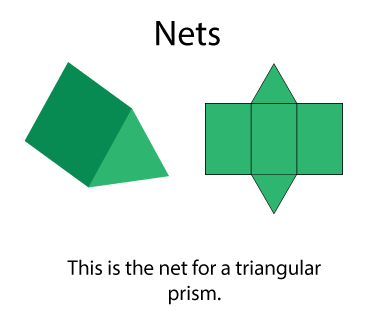This is the net for a square pyramid. Notice how the square base connects with the four triangular bases. This net will fold into a pyramid.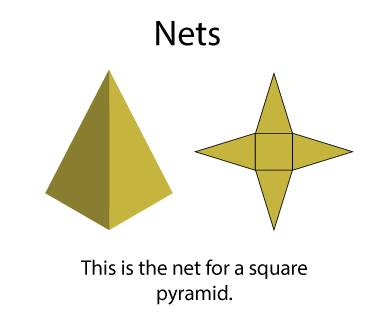Even circular figures can be modeled with a net. Here is the net for a cylinder. Notice how the circular the circular sides of the cylinder align with the rectangular side.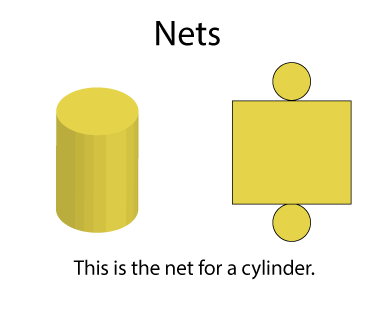This animated sequence shows a cube from top to bottom.